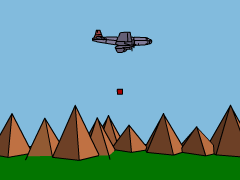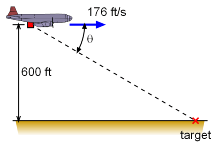Ch 1. Particle General Motion Multimedia Engineering Dynamics Position,Vel & Accel. Accel. varyw/ Time Accel. Constant Rect. Coordinates Norm/Tang. Coordinates Polar Coordinates RelativeMotion
 Chapter - Particle - 1. General Motion 2. Force & Accel. 3. Energy 4. Momentum - Rigid Body - 5. General Motion 6. Force & Accel. 7. Energy 8. Momentum 9. 3-D Motion 10. Vibrations Appendix Basic Math Units Basic Equations Sections Search eBooks Dynamics Fluids Math Mechanics Statics Thermodynamics Author(s): Kurt Gramoll ©Kurt GramollDYNAMICS - CASE STUDY IntroductionA cargo plane has to drop a relief package to people in need. How can the pilot determine when to drop the package? What is known: The plane is flying level (vertical velocity = 0) at 600 ft. The plane is traveling at 176 ft/s in a straight line toward the drop target. The wind resistance on the package can be neglected. QuestionProblem Diagram When releasing the package, at what angle θ down from the horizontal must the pilot see the target for the package to land on the target? Approach Use vectors to analyze curvilinear motion. Resolve the vectors for curvilinear motion into rectangular components. Integrate the acceleration to find the horizontal distance covered by the package, then use trigonometry to determine the angle θ.

Practice Homework and Test problems now available in the 'Eng Dynamics' mobile app
Includes over 400 problems with complete detailed solutions.
Available now at the Google Play Store and Apple App Store.